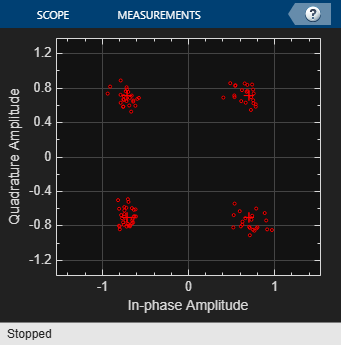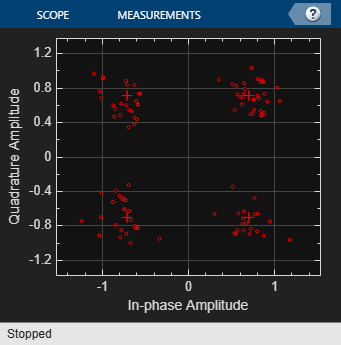# Random Integer Generator

Generate integers randomly distributed in specified range

•Libraries:
Communications Toolbox / Comm Sources / Random Data Sources

## Description

The Random Integer Generator block generates uniformly distributed random integers in the range [0, M-1], where M is specified by the Set size parameter. Use this block to generate random binary-valued or integer-valued data.

To ensure that the model uses different initial seeds, set the Simulate using parameter to `Interpreted execution`, and run the simulation in Normal or Accelerator mode. For more information, see Limitations.

## Examples

expand all

Demonstrate that the `Random Integer Generator` sequences output when the initial seed source is `Auto` are different and that when the initial seed source is `Parameter` the sequences are repeatable when the seed is not changed.Run the `slex_rng_seed_source` model twice and compare the output sequence.

``` 6 1 7 7 1 7 7 3 5 6 0 1 2 3 4 7 7 6 7 7 ```

Change the `Initial seed source` to `Parameter`. Run the `slex_rng_seed_source` model twice with the seed value set to `0` and compare the output sequence.

``` 6 6 7 7 1 1 7 7 5 5 0 0 2 2 4 4 7 7 7 7 ```

Change seed value to `1993`, rerun, and compare sequence to the previous sequences.

``` 6 6 0 7 7 1 1 1 7 7 7 2 5 5 1 0 0 1 2 2 4 4 4 5 7 7 4 7 7 1 ```

Apply QPSK modulation to a signal of random data. Pass the modulated signal through an AWGN channel. Plot the signal constellation.

The `doc_qpsk_mod` model generates QPSK data, applies white noise, and displays the resulting constellation diagram.Run the model with the Eb/N0 of the AWGN Channel block set to 15db.Change the Eb/N0 from 15 dB to 10 dB. The noise level increases as shown by the greater distance between the samples.The `cm_ex_viterbi_decode_binary_seq` model generates a binary sequence using the `Random Integer Generator` block. The sequence is encoded with the `Convolutional Encoder` block and then impaired with the `Binary Symmetric Channel` block. The `Viterbi Decoder` block decodes the data sequence and the bit error rate is computed.

The `InitFcn` callback is used to initialize workspace parameters for samples per frame, BSC error probability, and the Viterbi decoder traceback depth. The signal delay between the transmitted and received signal is equal to the traceback depth. The signal delay is needed for the error rate calculation.To produce a binary bit stream, the `Random Integer Generator` block specifies a set size of `2`, and output type of `boolean`.

The computed error rate approximates the `Error probability` specified in the `Binary Symmetric Channel` block.

```Computed error rate = 0.095023 ```

## Limitations

• In Rapid Accelerator simulation mode, when you set Simulate using to `Interpreted execution` and Source of initial seed to `Auto`, the block generates the same numbers every time the simulation runs. This behavior is equivalent to setting Source of initial seed to `Parameter` and setting Initial seed to `0`.

• In all simulation modes (Normal, Accelerator, and Rapid Accelerator), when you set Simulate using to `Code generation` and Source of initial seed to `Auto`, the block generates the same numbers every time the simulation runs. This behavior is equivalent to setting Source of initial seed to `Parameter` and Initial seed to `0`.

## Ports

### Output

expand all

Random integer output, returned as a scalar, vector, or matrix. This port is unnamed on the block. The data type is set using the Output data type parameter.

The number of rows in the output data equals the value of the Samples per frame parameter and corresponds to the number of samples in one frame. The number of columns in the output data equals the number of elements in the Set size parameter and corresponds to the number of channels.

## Parameters

expand all

To edit block parameters interactively, use the Property Inspector. From the Simulink® Toolstrip, on the Simulation tab, in the Prepare gallery, select .

Set size,M, specified as a positive integer or row vector of positive integers. The block generates integers in the range [0, (M – 1)]. The number of elements in Set size corresponds to the number of independent channels output from the block.

• If Set size is a scalar, then all output random variables are independent and identically distributed (i.i.d.).

• If Set size is a vector, then the length of the vector determines the number of output channels. The channels can have differing output ranges.

Source of the initial seed for the random number generator, specified as either:

• `Auto` –– the block uses the global random number stream

• `Parameter` –– the block sets the random number generator seed to Initial seed

Initial seed value for the random number generator, specified as a nonnegative integer. If the Initial seed parameter is a constant, then the resulting sequence is repeatable.

#### Dependencies

To enable this parameter, set the Source of initial seed parameter to `Parameter`.

Positive scalars specify the time in seconds between each sample of the output signal. If you set the Sample time to `-1`, the output signal inherits the sample time from downstream. For information on the relationship between the Sample time and Samples per frame parameters, see Sample Timing.

Samples per frame, specified as a positive integer indicating the number of samples per frame in one channel of the output data. For information on the relationship between Sample time and Samples per frame, see Sample Timing.

Output data type, specified as `double`, `single`, `uint8`, `uint16`, `uint32`, or `boolean`. If this parameter is set to `boolean`, you must set the Set size parameter to `2`.

Type of simulation to run, specified as:

• `Code generation` –– Simulate the model using generated C code. The first time you run a simulation, Simulink generates C code for the block. The C code is reused for subsequent simulations as long as the model does not change. This option requires additional startup time.

• `Interpreted execution` –– Simulate the model using the MATLAB® interpreter. This option shortens startup time. In `Interpreted execution` mode, you can debug the source code of the block.

## Block Characteristics

 Data Types `Boolean` | `double` | `integer` | `single` Multidimensional Signals `no` Variable-Size Signals `no`

expand all

## Version History

Introduced before R2006a

expand all